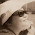## Saturday, 21 September 2013

### Revision for End-of-Year Exam (Maths)

Dear S1-07

With the end-of-year exam just a week away, most of you would have started revision.
We shall allocate at least one period (i.e. the last period to answer to enquiries).
To make the session really useful to you, we can do the following:

1. Go through the Exam Preparation Booklet - in particular the past year Test/ Exam papers.
2. Identify question(s) that you think would be helpful for class discussion.
3. You can post the question in the "Comment" of this post (e.g. 2012 Sec 1 Level Test 3 Q1).
4. I'll sort out the questions for discussion at the end of the week.

1.Ms Loh, There is a question in the Exam preparation booklet. It is in the topic of algebra. I'm not sure what the question number is, but it is between the 4th and the 10th question. It says that there is a wood plank 200cm, the person divided it into 44 pieces.The rods are either 4cm or 5cm long.Find the number of 4cm rods and 5 cm rods?May I know the method to find the answer?Because all I can do is guess and check and I think the method has something to do with algebra.

1.Taufiq

Let me know the page number so that I can locate the question.

2.Page No. 9, Question 6

3.The first line tells us that there are 44 pieces.
Since there are only 2 types of wood - either 5 cm long or 4 cm long.
Let the number of pieces that are 4 cm long be x.
Now the number of pieces that are 5 cm long will be 44-x. [agree?]

Since the length of the two types of woods are given in 'cm'.
It would be easier to convert the original "2 metres" be converted to "cm", which now becomes 200 cm.

Now, if we piece all the pieces of wood together,
what's the length made up by the 4 cm wood, and the length made up by the 5 cm wood?

OK, at this stage, are you able to use the above information to form an equation to solve for x?

:)

4.Ohhhhhh. I get it. 4x cm + 5(44 - x)cm = 200cm.
4x cm + 220 cm - 5x cm = 200cm
220 - x = 200
x = 20;

2.For the previous EOY, there is this question that has to do with polygons. I am able to complete it using quadratic equation. But is there a better way? It is question no. 9.

1.According to the question, there are 2 polygons.
So, if regular polygon B has N sides, then the regular polygon A will have N+5 sides.

Now, if interior angle of Polygon A is 3.6 degrees more than that of Polygon B
For Polygon B,
Let the interior angle be x, then exterior angle of 180-x
For Polygon A,
Interior angle of Polygon A = x + 3.6, then exterior angle = 180-(x+3.5)

For Polygon A, size of each exterior angle = 360/(N+5)
For Polygon B, size of each exterior angle = 360/N

By equating the above, we can form 2 equations:
For Polygon A, 180-(x+3.5) = 360/(N+5)
For Polygon B, 180-x = 360/N

Solve these 2 equations to find N and x.

3.Ms Loh, may I ask if you could help go through a bit of factorisation to refresh our memories, or mine at the very least. It will be much appreciated.

4.Ms Loh, for the Clementi town paper given. Question number 3 paper 1 :
the answer given is x:y is 10:7
but i got my answer as x:y is 7:10